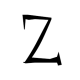Karl Patrik Johansson

Member

5

136 Neutral

• Rank
Newbie

• Website
• Interests
Art
Design
Programming

Social

zerratar
• Github
zerratar
• Twitch
zerratar
• Steam
zerratar
1. Hey frob!  Thank you for your reply! I must agree, this does aim at game development in Unity in general and not really specifically for just Android! So these apply in all cases ;-)   I've been using Unity now for almost 3 years and I've enjoyed every single bit of it. But I've also learned incredibly much during this period. I was hoping that this would help out others that was encountering the same issues I once did. /Karl
2. Hey guys!   I recently wrote an article on how I tackled my performance issues while i was developing my Android game Sky Blocks. I was hoping that this article would help you guys to get some insight when developing for android or any other mobile devices using Unity. I also want to see what you guys think about this article as well, critique and feedback are all welcome :-)   http://www.codeandux.com/tackling-performance-issues-in-unity-for-mobiles/ Best regards, Karl / Zerratar
3. Well, if you simply want to rotate the arm around its shoulder. You can use the following: var m = Mouse.GetState(); Vector2 mousePos = new Vector2(m.X, m.Y); Vector2 distance = mousePos - p1; // calculate angle to rotate float angle = (float)Math.Atan2(distance.Y, distance.X); spriteBatch.Begin(); spriteBatch.Draw(armAndGunTexture, p1.X, p.Y, null, Color.White, angle, Vector2.Zero,1f, SpriteEffects.None, 0); spriteBatch.End(); I hope this helped :)
4. Hurray! I'm glad it worked for you! :)
5. Hi football94! You can calculate the rotation between the two world points (Vector3)  This is what I used in my game: public Quaternion GetRotation(Vector3 targetPos) { return GetRotation(this.Position, targetPos); } public Quaternion GetRotation(Vector3 entityPos, Vector3 targetPos) { // the new forward vector, so the avatar faces the target Vector3 newForward = Vector3.Normalize(entityPos - targetPos); // calc the rotation so the avatar faces the target var src = Vector3.Forward; var dest = newForward; src.Normalize(); dest.Normalize(); float d = Vector3.Dot(src, dest); if (d >= 1f) { return Quaternion.Identity; } if (d < (1e-6f - 1.0f)) { Vector3 axis = Vector3.Cross(Vector3.UnitX, src); if (axis.LengthSquared() == 0) { axis = Vector3.Cross(Vector3.UnitY, src); } axis.Normalize(); return Quaternion.CreateFromAxisAngle(axis, MathHelper.Pi); } float s = (float)Math.Sqrt((1 + d) * 2); float invS = 1 / s; Vector3 c = Vector3.Cross(src, dest); Quaternion q = new Quaternion(invS * c, 0.5f * s); q.Normalize(); return q; } when drawing your model use: NewPosition = should be the target (future) position that your model is moving towards. Quaternion RotationQuat = GetRotation(NewPosition);   then you need to store your RotationQuat and do NOT update it until your new "future" position is changed. otherwise the model will rotate back the moment you stop moving. for (int i = 0; i < bones.Length; i++) { bones[i] *= Matrix.CreateFromQuaternion(RotationQuat) * Matrix.CreateTranslation(position) * Matrix.CreateWorld(Translation, Vector3.Forward, Vector3.Up); //Move the models position ... I hope this helped :)